﻿ 关于JS中的作用域中的问题思考分享 - 自由资讯

# 关于JS中的作用域中的问题思考分享

• 作用域
• 全局作用域
• 作用域中的错误
• 局部作用域
• with
• 弊端
• 数据泄露
• 性能下降
• let
• const
• 作用域链
• 闭包
• 闭包对作用域链的影响
• 匿名函数的赋值
• 使用let

## 全局作用域

```function test(a){
var b = a * 2
function test2(c){
console.log(a ,b, c)
}
test2(b * 3)
}
test(4) // 4 8 24
```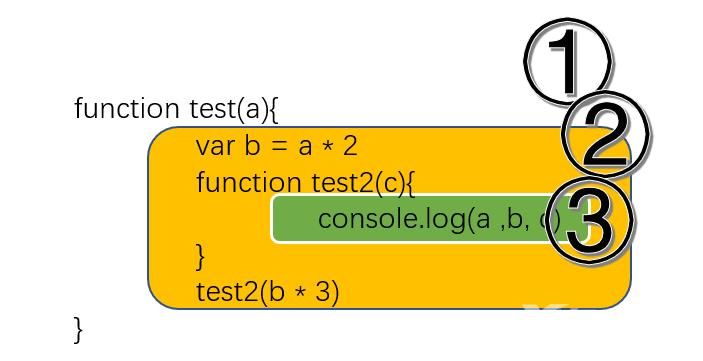• ①对应着全局` scope`，这里只有` test2`
• ②是` test2`界定的作用域，包含a、b、bar
• ③是bar界定的作用域，这里只有c这个变量。

### 作用域中的错误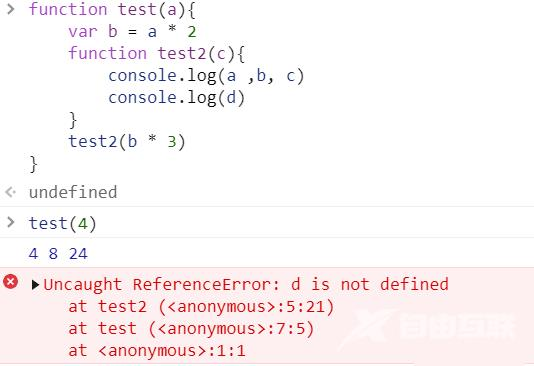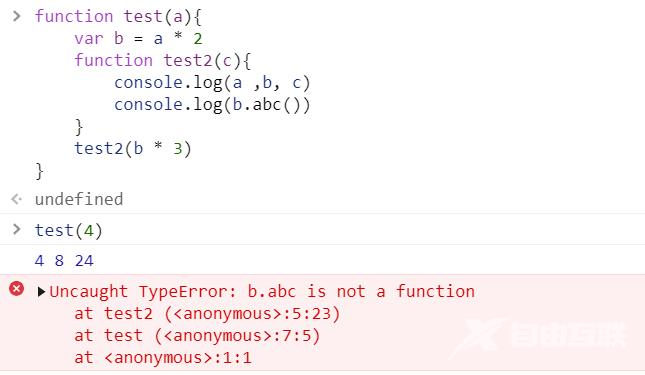## 局部作用域

### with

`with`语句的原本用意是为逐级的对象访问提供命名空间式的速写方式，也就是说在指定的代码区域，直接通过节点名称调用对象。` with`通常被当做重复引用同一个对象中的多个属性的快捷方式，可以不需要重复引用对象本身。如下面代码

```var obj = {a: 2, b: 2, c: 2};
with (obj) {
a = 5;
b = 5;
c = 5;
}
console.log(obj) // {a: 5, b: 5, c: 5}
```

• 数据泄露
• 性能下降

### 数据泄露

```function test3(obj) {
with (obj) {
a = 2;
}
}

var o1 = {
a: 3
};

var o2 = {
b: 3
}

foo(o1);
console.log(o1.a)

foo(o2);
console.log(o2.a);
console.log(a);
```### 性能下降

with 会在运行时修改或创建新的作用域，以此来欺骗其他在开发时定义的词法作用域。with的使用可以令代码更具有扩展性，虽然有数据泄漏的可能，但只要稍加注意就可以避免，除此之后，灵活运用难道不可以创造出很好地功能吗？事实上真的不能，不妨我们考察一下性能特点

```function test4() {
console.time("test4");
var obj = {
a: [1, 2, 3]
};
for(var i = 0; i < 100000; i++)
{
var v = obj.a;
}
console.timeEnd("test4");
}
test4();

function testWith() {
console.time("testWith");
var obj = {
a: [1, 2, 3]
};
with(obj) {
for(var i = 0; i < 100000; i++) {
var v = a;
}
}
console.timeEnd("testWith");
}

testWith();
```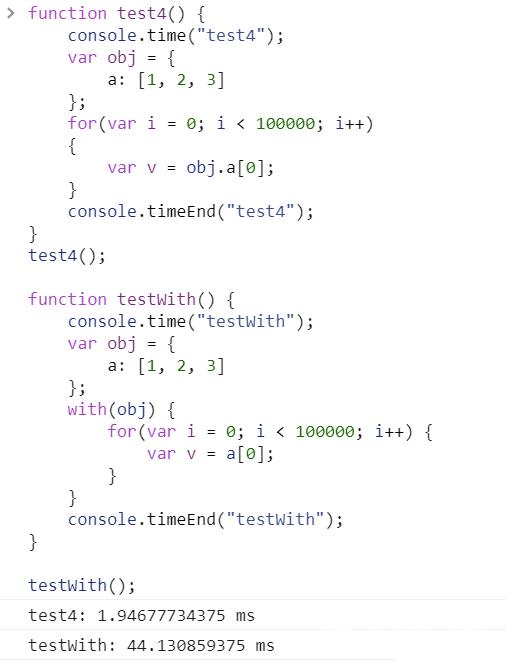### let

```var test5 = true;
if (test5) {
let bar = test5 * 2;
console.log( bar );
}
console.log( bar ); // ReferenceError
```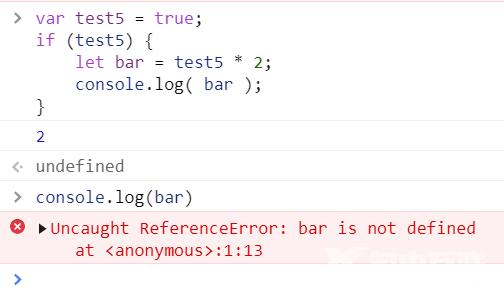### const

```var test6 = true;
if (test6) {
var a = 2;
const b = 3;
a = 3;
b = 4;
}
console.log( a );
console.log( b );
```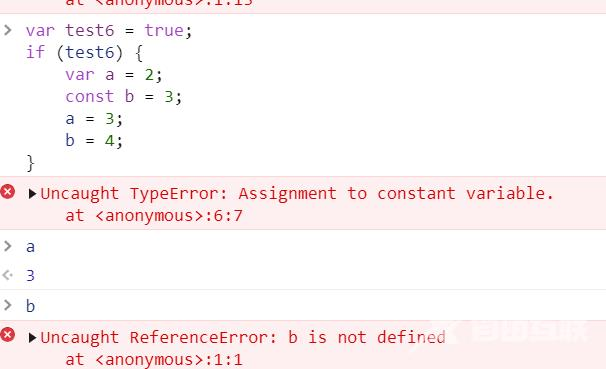## 作用域链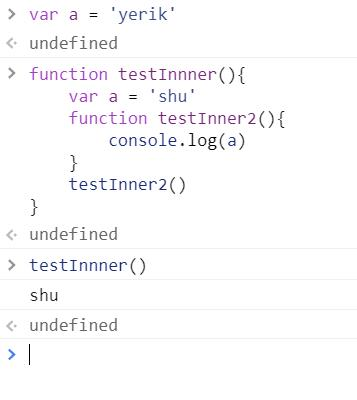### 闭包

```function test7() {
var a = 2;
function test8() {
console.log( a ); // 2
}
test8();
}
test7();
```

```function test7() {
var a = 2;
function test8() {
console.log( a ); // 2
}
return test8;
}
var test9 = test7();
test9(); // 2
```

```function createClosure(){
var name = "yerik";
return {
setStr:function(){
name = "naug";
},
getStr:function(){
return name + ":hello";
}
}
}
var builder = new createClosure();
builder.setStr();
console.log(builder.getStr());
```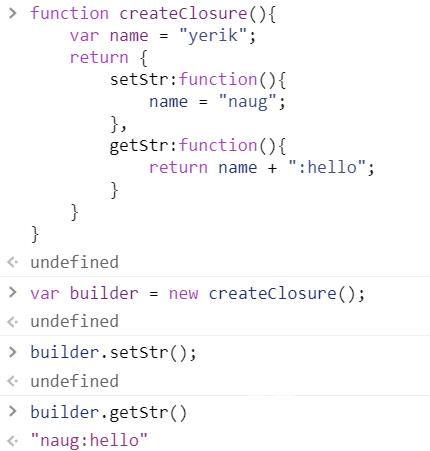### 闭包对作用域链的影响

```var data = [];

for (var i = 0; i < 3; i++) {
data[i] = function () {
console.log(i);
};
}
console.log(data)
console.log(data)
console.log(data)
```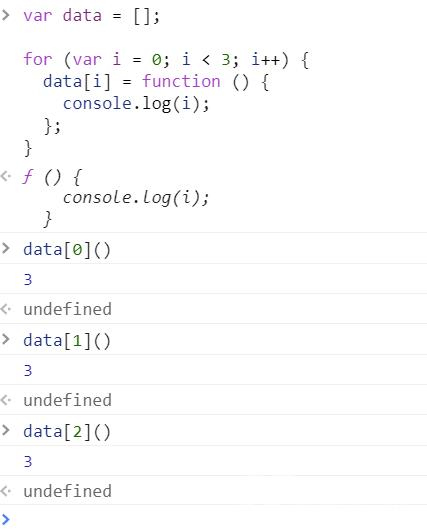• 返回匿名函数的赋值或者立即执行函数
• 使用es6的let

### 匿名函数的赋值

```var data = [];

for (var i = 0; i < 3; i++) {
data[i] = (function (num) {
return function(){
console.log(num);
}
})(i);
}
console.log(data)
console.log(data)
console.log(data)
```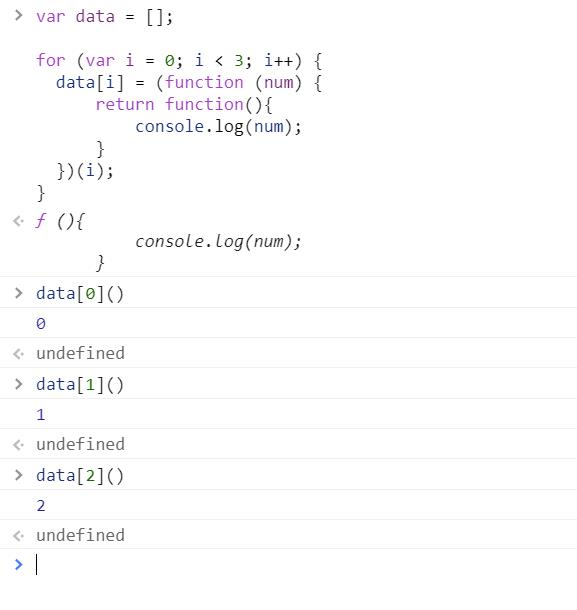### 使用let

```var data = [];

for (let i = 0; i < 3; i++) {
data[i] = (function (num) {
return function(){
console.log(num);
}
})(i);
}
console.log(data)
console.log(data)
console.log(data)
```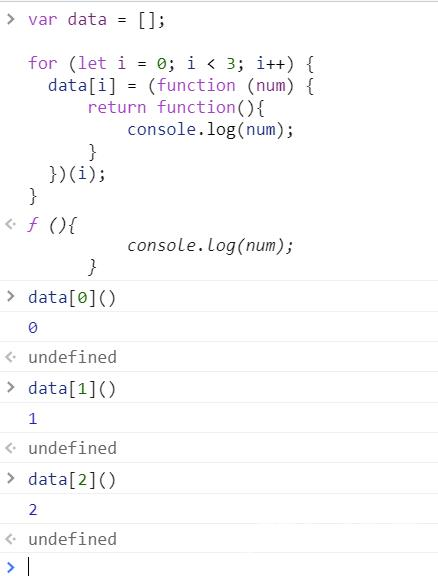```var data = [];// 创建一个数组data;

{
// 进入第一次循环
let i = 0; // 注意：因为使用let使得for循环为局部作用域
// 此次 let i = 0 在这个局部作用域中，而不是在全局环境中
data = function() {
console.log(i);
};
}
{
// 进入第二次循环
let i = 1; // 因为 let i = 1 和上面的 let i = 0
// 在不同的作用域中，所以不会相互影响
data = function(){
console.log(i);
};
}
...
```

```{
let i = 1;
data = function(){
console.log(i);
};
}
```

﻿